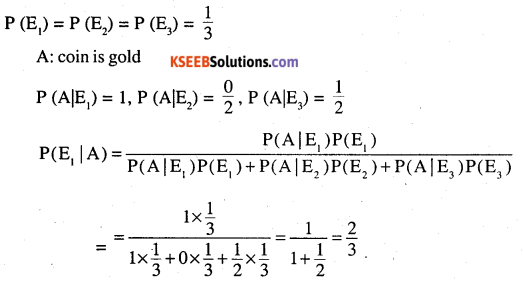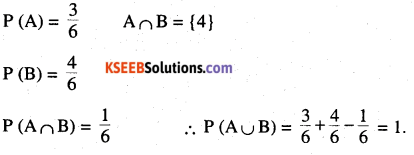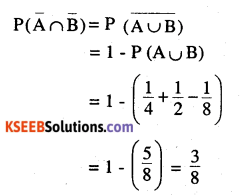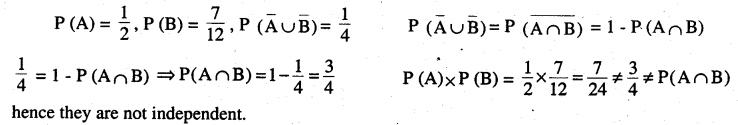# 2nd PUC Maths Question Bank Chapter 13 Probability Miscellaneous Exercise

Students can Download Maths Chapter 13 Probability Ex 13.4 Questions and Answers, Notes Pdf, 2nd PUC Maths Question Bank with Answers helps you to revise the complete Karnataka State Board Syllabus and score more marks in your examinations.

## Karnataka 2nd PUC Maths Question Bank Chapter 13 Probability Miscellaneous Exercise

Question 1.
A family has two children. Find the probability that both are boys, if it is known that
(i) At least one of them is boy
(ii) Elder child is a boy (CBSE – 2010)
(i) Let A : At least one of them is a boy
{BB,BG,GB,GG}Question 2.
A bag contains 4 balls
Two balls are drawn at random and are found to be White. What is the probability that all Balls are white.
E1: 2 balls are white, 2- are non white
E2: 3 balls are white, 1 is non white
E3: 4 balls are white
A : Two balls are whiteQuestion 3.
Given three identical boxes I, II and III each containing two coins. In box I, both coins are gold, In box II both are silver and in box III there is one gold and one silver coin. A person choose a box and takes out a coin. If the coin is gold, what is the probability that the other coin is also gold  (CBSE – 2011)
E1: Both gold coin
E2: Both silver coin
E3: One gold and one silverQuestion 4.
Two cards are drawn without replacement from a well shuffled pack of cards. Find the mean and variance of the number of Red cards.  (CBSE – 2012)
Let X denote the no. of Red cardsQuestions from Competitive Exams

Question 1.
A die is thrown. Let A be the event that the number obtained: is greater than 3. B be the event that the number obtained is less than 5. Find P(A∪B)Question 2.
It is given that, the events A and B are such that
$$\mathbf{P}(\mathbf{A})=\frac{1}{4}, \mathbf{P}(\mathbf{A} | \mathbf{B})=\frac{1}{2}, \mathbf{P}(\mathbf{B} | \mathbf{A})=\frac{2}{3}, \text { Find } P(B)$$ [AIEEE – 2008]Question 3.
$$\mathbf{P}(\mathbf{A})=\frac{1}{4}, \mathbf{P}(\mathbf{B})=\frac{1}{2}, \mathbf{P}(\mathbf{A} \cap \mathbf{B})=\frac{1}{8}$$,find P(not A, not B) (UPSEE -2008)